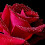## Page

### Difference Between Wattmeter and Energy Meter

The main comparison between wattmeter and energy meter is that the wattmeter measures the electrical power flows in the circuit in watt while the energy meter measures the total electrical energy consumed by the electrical load. Here this article give information about the key difference between wattmeter and energy meter are listed below.

Definition of wattmeter:

The wattmeter is an instrument for measuring the electric power flow in a given circuit in watt. The internal construction of a wattmeter is such that it consists of using two coils. One of the coils is in series and the other is connected in parallel. The coil that is connected in series with the circuit which is known as the current coil and the one that is connected in parallel with the circuit which is known as the voltage coil.

Definition of energymeter:

The meter is used for measuring the energy utilizes by the electrical load known as the energy meter. The energy is the total power consumed and utilized by the load at a particular interval time. It is also used in domestic and industrial AC circuit for measuring the power consumption. The meter leads expensive and accurate.

The main key difference between wattmeter and energy meter are listed below:

• The energy meter measures the total energy consumed by the load while the wattmeter measures the power in a circuit.
• The energy meter measures the energy in joules but wattmeter the measure the power in watts.
• The energy meter driving, braking, and moving system along with the counting mechanism are the main parts, but the wattmeter pressure and current coil, control system, scale and pointer, damping system to the central part.
• The energy meter work on the principle of conversion of electrical energy into mechanical energy.  Whereas the wattmeter works on the principle that the force acts on the current-carrying conductor when it is placed in an electromagnetic field.
• The energy meter is used for measuring the total power consumed by the load in the industries and homes while the wattmeter is used for measuring the power of an electrical circuit. It is also used for determining the power rating of the homes and industrial appliances.
The main comparison between wattmeter and energy meter is that the wattmeter measures the electrical power flows in the circuit in watt while the energy meter measures the total electrical energy consumed by the electrical load. Here this article give information about the key difference between wattmeter and energy meter are listed below.

Definition of wattmeter:

The wattmeter is an instrument for measuring the electric power flow in a given circuit in watt. The internal construction of a wattmeter is such that it consists of using two coils. One of the coils is in series and the other is connected in parallel. The coil that is connected in series with the circuit which is known as the current coil and the one that is connected in parallel with the circuit which is known as the voltage coil.

Definition of energymeter:

The meter is used for measuring the energy utilizes by the electrical load known as the energy meter. The energy is the total power consumed and utilized by the load at a particular interval time. It is also used in domestic and industrial AC circuit for measuring the power consumption. The meter leads expensive and accurate.

The main key difference between wattmeter and energy meter are listed below:

• The energy meter measures the total energy consumed by the load while the wattmeter measures the power in a circuit.
• The energy meter measures the energy in joules but wattmeter the measure the power in watts.
• The energy meter driving, braking, and moving system along with the counting mechanism are the main parts, but the wattmeter pressure and current coil, control system, scale and pointer, damping system to the central part.
• The energy meter work on the principle of conversion of electrical energy into mechanical energy.  Whereas the wattmeter works on the principle that the force acts on the current-carrying conductor when it is placed in an electromagnetic field.
• The energy meter is used for measuring the total power consumed by the load in the industries and homes while the wattmeter is used for measuring the power of an electrical circuit. It is also used for determining the power rating of the homes and industrial appliances.

1.oprah also makes some good book reviews, i always wait for the book reviews of oprah. Flow Meter LC

2.I’m happy I located this blog! From time to time, students want to cognitive the keys of productive literary essays composing. Your first-class knowledge about this good post can become a proper basis for such people. nice one fitness tracker

3.This is such a great resource that you are providing and you give it away for free. I love seeing blog that understand the value of providing a quality resource for free. hemp wellness

4.Nice reading, I love your content. This is really a fantastic and informative post. Keep it up and if you are looking for mission driving school then visit Maple Driving School.

5.Choosing the correct stream meter is a significant piece of synthetic handling. It will tell you whether your preparing gear works appropriately or there is an issue. With the right Chemical Flow Meter, you can gauge stream precisely and recognize whether there is any break in the pipelines. Also, these meters will tell you how much liquid you are utilizing at present. In a word; with the correct meter, you can deal with an exact cycle control.scientificdevices.org

6.Your post is very informative. Thanks for sharing.
MEPCO Duplicate Bill
Check 10 Incredible New Ways of Generating Electricity

7.This blog is amazing and great resource of information. Well explain about Wattmeter and Energy Meter.
mepcobillonline

8.Electricians are trained to interpret electrical codes and Heating and Cooling regulations specific to different industries.